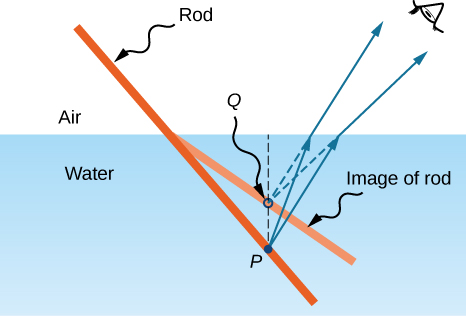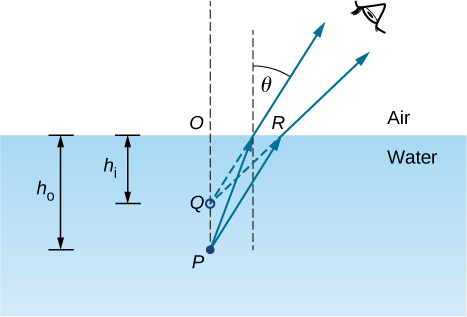# 2.3 Images formed by refraction

 Page 1 / 5
By the end of this section, you will be able to:
• Describe image formation by a single refracting surface
• Determine the location of an image and calculate its properties by using a ray diagram
• Determine the location of an image and calculate its properties by using the equation for a single refracting surface

When rays of light propagate from one medium to another, these rays undergo refraction, which is when light waves are bent at the interface between two media. The refracting surface can form an image in a similar fashion to a reflecting surface, except that the law of refraction (Snell’s law) is at the heart of the process instead of the law of reflection.

## Refraction at a plane interface—apparent depth

If you look at a straight rod partially submerged in water, it appears to bend at the surface ( [link] ). The reason behind this curious effect is that the image of the rod inside the water forms a little closer to the surface than the actual position of the rod, so it does not line up with the part of the rod that is above the water. The same phenomenon explains why a fish in water appears to be closer to the surface than it actually is.Bending of a rod at a water-air interface. Point P on the rod appears to be at point Q , which is where the image of point P forms due to refraction at the air-water interface.

To study image formation as a result of refraction, consider the following questions:

1. What happens to the rays of light when they enter or pass through a different medium?
2. Do the refracted rays originating from a single point meet at some point or diverge away from each other?

To be concrete, we consider a simple system consisting of two media separated by a plane interface ( [link] ). The object is in one medium and the observer is in the other. For instance, when you look at a fish from above the water surface, the fish is in medium 1 (the water) with refractive index 1.33, and your eye is in medium 2 (the air) with refractive index 1.00, and the surface of the water is the interface. The depth that you “see” is the image height ${h}_{\text{i}}$ and is called the apparent depth    . The actual depth of the fish is the object height ${h}_{\text{o}}$ .Apparent depth due to refraction. The real object at point P creates an image at point Q . The image is not at the same depth as the object, so the observer sees the image at an “apparent depth.”

The apparent depth ${h}_{\text{i}}$ depends on the angle at which you view the image. For a view from above (the so-called “normal” view), we can approximate the refraction angle $\theta$ to be small, and replace sin $\theta$ in Snell’s law by tan $\theta$ . With this approximation, you can use the triangles $\text{Δ}OPR$ and $\text{Δ}OQR$ to show that the apparent depth is given by

${h}_{\text{i}}=\left(\frac{{n}_{2}}{{n}_{1}}\right){h}_{\text{o}}.$

The derivation of this result is left as an exercise. Thus, a fish appears at 3/4 of the real depth when viewed from above.

## Refraction at a spherical interface

Spherical shapes play an important role in optics primarily because high-quality spherical shapes are far easier to manufacture than other curved surfaces. To study refraction at a single spherical surface, we assume that the medium with the spherical surface at one end continues indefinitely (a “semi-infinite” medium).

What is photoelectric
If you lie on a beach looking at the water with your head tipped slightly sideways, your polarized sunglasses do not work very well.Why not?
it has everything to do with the angle the UV sunlight strikes your sunglasses.
Jallal
this is known as optical physics. it describes how visible light, ultraviolet light and infrared light interact when they come into contact with physical matter. usually the photons or light upon interaction result in either reflection refraction diffraction or interference of the light.
Jallal
I hope I'm clear if I'm not please tell me to clarify further or rephrase
Jallal
what is bohrs model for hydrogen atom
hi
Tr
Hello
Youte
Hi
Nwangwu-ike
hi
Siddiquee
hi
Omar
helo
Mcjoi
what is the value of speed of light
1.79×10_¹⁹ km per hour
Swagatika
what r dwarf planet
what is energy
কাজের একক কী
Jasim
কাজের একক কী
Jasim
friction ka direction Kaise pata karte hai
friction is always in the opposite of the direction of moving object
Punia
A twin paradox in the special theory of relativity arises due to.....? a) asymmetric of time only b) symmetric of time only c) only time
b) symmetric of time only
Swagatika
fundamental note of a vibrating string
every matter made up of particles and particles are also subdivided which are themselves subdivided and so on ,and the basic and smallest smallest smallest division is energy which vibrates to become particles and thats why particles have wave nature
Alvin
what are matter waves? Give some examples
according to de Broglie any matter particles by attaining the higher velocity as compared to light'ill show the wave nature and equation of wave will applicable on it but in practical life people see it is impossible however it is practicaly true and possible while looking at the earth matter at far
Manikant
a centeral part of theory of quantum mechanics example:just like a beam of light or a water wave
Swagatika
Mathematical expression of principle of relativity
given that the velocity v of wave depends on the tension f in the spring, it's length 'I' and it's mass 'm'. derive using dimension the equation of the wave
What is the importance of de-broglie's wavelength?
he related wave to matter
Zahid
at subatomic level wave and matter are associated. this refering to mass energy equivalence
Zahid
it is key of quantum
Manikant
how those weight effect a stable motion at equilibrium
how do I differentiate this equation- A sinwt with respect to t
just use the chain rule : let u =wt , the dy/dt = dy/du × du/dt : wA × cos(wt)
Jerry
I see my message got garbled , anyway use the chain rule with u= wt , etc...
JerryBy Anh DaoBy Stephen VoronBy OpenStaxBy OpenStaxBy OpenStaxBy Richley CrapoBy OpenStaxBy Stephen VoronBy Jams KaloBy John Gabrieli